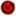### Simple Blocks

Blocks are Glee's stored program elements. Everything between curly braces ({...}) Glee takes as code for the block. Blocks in Glee are objects just like other Glee elements. Code is just one of a block's several members. Others are its namespace, its initial expression, its break expression, and its error expression. For a general description of this tutorial, press.Code Result{'hello world'} Result:
hello world"{'hello world'}" #pgm =>hw;
hw \$;
hello world'<1>---------' \$;
'HW'#pgm {'hello world'} %** \$;
'<2>---------' \$;
HW %** \$;
'<3>---------' \$;
HW: %** \$;
'<4>---------' \$;
HW:code %** \$;
'<5>---------' \$;
HW:string \$;
'<6>---------' \$;
HW:stringv \$;
'<7>---------' \$;
HW:obj %** \$;
<1>---------
String[R2C2:C]HW
<2>---------
String[R2C11:C]hello world
<3>---------
String[R2C61:C]:, :code, :ns, :obj, :size, :string, :stringv, :typec, :typen
<4>---------
String[R2C15:C]{'hello world'}
<5>---------
Blk:(Pgm:C:{'hello world'})
<6>---------
Pgm[R2C1:K]
Code:
{'hello world'}
<7>---------
Pgm[R3C1:K]
Code:
{'hello world'}'HW'#pgm {'hello world'} ;
'<1>---------' \$;
'This is the ' (HW) ' program' \$;
'<2>---------' \$;
'This is the ' (HW) ' program' %** \$;
'<3>---------' \$;
'This is the ' HW ' program' %** \$;
<1>---------
This is the hello world program
<2>---------
Seq[R1C3:K]
String[R1C12:C]This is the
String[R1C11:C]hello world
String[R2C8:C] program
<3>---------
Seq[R1C3:K]
String[R1C12:C]This is the
String[R1C11:C]hello world
String[R2C8:C] program'HW'#pgm{arg ' hello world '};
'<1>-----------' \$;
HW:stringv \$;
'<2>-----------' \$;
HW:ns %**\$;
'<3>-----------' \$;
'this is the '[.arg]HW ' program' %** \$;
'<4>-----------' \$;
HW:ns %**\$;
'<5>-----------' \$;
HW ' again' %** \$;
'<5>-----------' \$;
'this was the' HW ' again' %** \$;
'<6>-----------' \$;
'this was the'[.arg] HW ' again' %** \$;
'<7>-----------' \$;
'this was the'[.xyz] HW ' again' %** \$;
'<8>-----------' \$;
HW:ns %**\$;
<1>-----------
Pgm[R2C1:K]
Code:
{arg ' hello world '}
<2>-----------
NS[R2C0:K]

<3>-----------
Seq[R1C3:K]
String[R3C12:C]this is the
String[R1C13:C] hello world
String[R2C8:C] program
<4>-----------
NS[R2C1:K]
Assoc[R2C1:P]:arg:String
<5>-----------
Seq[R1C3:K]
String[R3C12:C]this is the
String[R1C13:C] hello world
String[R2C6:C] again
<5>-----------
Seq[R1C3:K]
String[R1C12:C]this was the
Seq[R1C2:K]
*String[R3C12:C]this is the
*String[R1C13:C] hello world
String[R2C6:C] again
<6>-----------
Seq[R1C3:K]
String[R3C12:C]this was the
String[R1C13:C] hello world
String[R2C6:C] again
<7>-----------
Seq[R1C3:K]
String[R2C12:C]this was the
String[R1C13:C] hello world
String[R2C6:C] again
<8>-----------
NS[R2C2:K]
Assoc[R2C1:P]:arg:String
Assoc[R2C1:P]:xyz:String#ns => @;
'HW'#pgm{#ns => @;arg ' hello world '};
'<1>------------' \$;
'this is the '[.arg]HW ' program' %** \$;
'<2>------------' \$;
HW ' again' %** \$; 'arg in root '=>arg;
'<3>------------' \$;
'this is the '[.arg]HW ' program' %** \$;
'<4>------------' \$;
HW ' again' %** \$;
<1>------------
Seq[R1C2:K]
String[R1C13:C] hello world
String[R2C8:C] program
<2>------------
Seq[R1C2:K]
String[R1C13:C] hello world
String[R2C6:C] again
<3>------------
Seq[R1C3:K]
String[R2C12:C]this is the
String[R1C13:C] hello world
String[R2C8:C] program
<4>------------
Seq[R1C3:K]
String[R2C12:C]this is the
String[R1C13:C] hello world
String[R2C6:C] again#ns => @;
'HW'#pgm{#ns => @;#args;arg ' hello world '};
'<1>------------' \$;
'this is the '[.arg]HW ' program' %** \$;
'<2>------------' \$;
HW ' again' %** \$; 'arg in root '=>arg;
'<3>------------' \$;
'this is the '[.arg]HW ' program' %** \$;
'<4>------------' \$;
HW ' again' %** \$;
<1>------------
Seq[R1C3:K]
NV:
String[R1C13:C] hello world
String[R2C8:C] program
<2>------------
Seq[R1C3:K]
NV:
String[R1C13:C] hello world
String[R2C6:C] again
<3>------------
Seq[R1C3:K]
String[R2C12:C]arg in root
String[R1C13:C] hello world
String[R2C8:C] program
<4>------------
Seq[R1C3:K]
String[R2C12:C]arg in root
String[R1C13:C] hello world
String[R2C6:C] again#ns => @;
'HW'#pgm{#ns => @;#args;
arg ' hello world '(#ns => @)->_1};
'<1>------------' \$;
'this is the '[.arg]HW ' program' %** \$;
'<2>------------' \$;
HW ' again' %** \$; 'arg in root '=>arg;
'<3>------------' \$;
'this is the '[.arg]HW ' program' %** \$;
'<4>------------' \$;
HW:ns %** \$;
<1>------------
Seq[R1C3:K]
String[R2C12:C]this is the
String[R1C13:C] hello world
String[R2C8:C] program
<2>------------
Seq[R1C3:K]
NV:
String[R1C13:C] hello world
String[R2C6:C] again
<3>------------
Seq[R1C3:K]
String[R2C12:C]this is the
String[R1C13:C] hello world
String[R2C8:C] program
<4>------------
NS[R2C0:K]